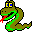#Boost C++ Libraries

...one of the most highly regarded and expertly designed C++ library projects in the world.#BFS Visitor Concept

This concept defines the visitor interface for breadth_first_search(). Users can define a class with the BFS Visitor interface and pass and object of the class to breadth_first_search(), thereby augmenting the actions taken during the graph search.

### Refinement of

Copy Constructible (copying a visitor should be a lightweight operation).

### Notation

 V A type that is a model of BFS Visitor. vis An object of type V. G A type that is a model of Graph. g An object of type G. e An object of type boost::graph_traits::edge_descriptor. s,u An object of type boost::graph_traits::vertex_descriptor.

none

### Valid Expressions

NameExpressionReturn TypeDescription
Initialize Vertex vis.initialize_vertex(s, g) void This is invoked on every vertex of the graph before the start of the graph search.
Discover Vertex vis.discover_vertex(u, g) void This is invoked when a vertex is encountered for the first time.
Examine Vertex vis.examine_vertex(u, g) void This is invoked on a vertex as it is popped from the queue. This happens immediately before examine_edge() is invoked on each of the out-edges of vertex u.
Examine Edge vis.examine_edge(e, g) void This is invoked on every out-edge of each vertex after it is discovered.
Tree Edge vis.tree_edge(e, g) void This is invoked on each edge as it becomes a member of the edges that form the search tree.
Non-Tree Edge vis.non_tree_edge(e, g) void This is invoked on back or cross edges for directed graphs and cross edges for undirected graphs.
Gray Target vis.gray_target(e, g) void This is invoked on the subset of non-tree edges whose target vertex is colored gray at the time of examination. The color gray indicates that the vertex is currently in the queue.
Black Target vis.black_target(e, g) void This is invoked on the subset of non-tree edges whose target vertex is colored black at the time of examination. The color black indicates that the vertex has been removed from the queue.
Finish Vertex vis.finish_vertex(u, g) void This invoked on a vertex after all of its out edges have been added to the search tree and all of the adjacent vertices have been discovered (but before the out-edges of the adjacent vertices have been examined).

### Python

To implement a model of the BFSVisitor concept in Python, create a new class that derives from the BFSVisitor type of the graph, which will be named GraphType.BFSVisitor. The events and syntax are the same as with visitors in C++. Here is an example for the Python bgl.Graph graph type:
```class count_tree_edges_bfs_visitor(bgl.Graph.BFSVisitor):
def __init__(self, name_map):
bgl.Graph.BFSVisitor.__init__(self)
self.name_map = name_map

def tree_edge(self, e, g):
(u, v) = (g.source(e), g.target(e))
print "Tree edge ",
print self.name_map[u],
print " -> ",
print self.name_map[v]
```